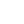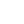Preview the Course
1h 1m

# Point Slope Form and Factoring Quadratics

by Juliet Goodman | Math FREE12 Lessons 1:01:12
1.1. Factoring Quadratics 4:34
2.2. Elimination Method 4:46
3.3. Point Slope 1 0:38
4.4. Point Slope 2 7:20
5.5. Quad Formula 13:29
6.6. Quadratics 1 7:46
7.7. Quadratics 2 1:25
8.8. Slope Intercept 1 7:47
9.9. Slope Intercept 2 6:20
10.10. Substitution Method 4:02
11.11. Systems 1 0:32
12.12. Systems 2 2:33

1h 1m

NGSS Standards

FREE

Course

We will learn about point slope form and factoring quadratics. Understanding the basics of algebra is crucial to grasp more the advanced topics in mathematics that will arise as you progress through school. Professionals in fields like engineering and finance utilize these foundational ideas and the topics that build on them alike. This course explains various ways to solve and understand miscellaneous topics such as lines, systems of linear equations, and quadratic equations.

Tags:

• Equations
• Graphs
• Lines
• Math